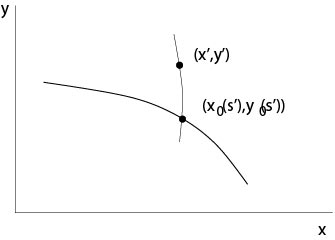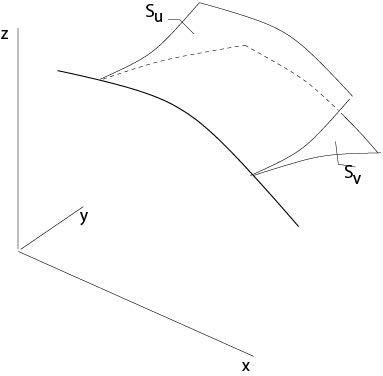$$\newcommand{\id}{\mathrm{id}}$$ $$\newcommand{\Span}{\mathrm{span}}$$ $$\newcommand{\kernel}{\mathrm{null}\,}$$ $$\newcommand{\range}{\mathrm{range}\,}$$ $$\newcommand{\RealPart}{\mathrm{Re}}$$ $$\newcommand{\ImaginaryPart}{\mathrm{Im}}$$ $$\newcommand{\Argument}{\mathrm{Arg}}$$ $$\newcommand{\norm}{\| #1 \|}$$ $$\newcommand{\inner}{\langle #1, #2 \rangle}$$ $$\newcommand{\Span}{\mathrm{span}}$$

# 2.2.2: Initial Value Problem of Cauchy

$$\newcommand{\vecs}{\overset { \rightharpoonup} {\mathbf{#1}} }$$ $$\newcommand{\vecd}{\overset{-\!-\!\rightharpoonup}{\vphantom{a}\smash {#1}}}$$$$\newcommand{\id}{\mathrm{id}}$$ $$\newcommand{\Span}{\mathrm{span}}$$ $$\newcommand{\kernel}{\mathrm{null}\,}$$ $$\newcommand{\range}{\mathrm{range}\,}$$ $$\newcommand{\RealPart}{\mathrm{Re}}$$ $$\newcommand{\ImaginaryPart}{\mathrm{Im}}$$ $$\newcommand{\Argument}{\mathrm{Arg}}$$ $$\newcommand{\norm}{\| #1 \|}$$ $$\newcommand{\inner}{\langle #1, #2 \rangle}$$ $$\newcommand{\Span}{\mathrm{span}}$$ $$\newcommand{\id}{\mathrm{id}}$$ $$\newcommand{\Span}{\mathrm{span}}$$ $$\newcommand{\kernel}{\mathrm{null}\,}$$ $$\newcommand{\range}{\mathrm{range}\,}$$ $$\newcommand{\RealPart}{\mathrm{Re}}$$ $$\newcommand{\ImaginaryPart}{\mathrm{Im}}$$ $$\newcommand{\Argument}{\mathrm{Arg}}$$ $$\newcommand{\norm}{\| #1 \|}$$ $$\newcommand{\inner}{\langle #1, #2 \rangle}$$ $$\newcommand{\Span}{\mathrm{span}}$$

Consider again the quasilinear equation

($$\star$$) $$a_1(x,y,u)u_x+a_2(x,y,u)u_y=a_3(x,y,u)$$.

Let
$$\Gamma:\ \ x=x_0(s),\ y=y_0(s),\ z=z_0(s), \ s_1\le s\le s_2,\ -\infty<s_1<s_2<+\infty$$
be a regular curve in $$\mathbb{R}^3$$ and denote by $$\mathcal{C}$$ the orthogonal projection of $$\Gamma$$ onto the $$(x,y)$$-plane, i. e.,
$$\mathcal{C}:\ \ x=x_0(s),\ \ y=y_0(s).$$

Initial value problem of Cauchy: Find a $$C^1$$-solution $$u=u(x,y)$$ of $$(\star)$$ such that $$u(x_0(s),y_0(s))=z_0(s)$$, i. e., we seek a surface $$\mathcal{S}$$ defined by $$z=u(x,y)$$ which contains the curve $$\Gamma$$.Figure 2.2.2.1: Cauchy initial value problem

Definition. The curve $$\Gamma$$ is said to be non-characteristic if
$$x_0'(s)a_2(x_0(s),y_0(s))-y_0'(s)a_1(x_0(s),y_0(s))\not=0.$$

Theorem 2.1. Assume $$a_1,\ a_2,\ a_2\in C^1$$ in their arguments, the initial data $$x_0,\ y_0,\ z_0\in C^1[s_1,s_2]$$ and $$\Gamma$$ is non-characteristic.

Then there is a neighborhood of $$\cal{C}$$ such that there exists exactly one solution $$u$$ of the Cauchy initial value problem.

Proof. (i) Existence. Consider the following initial value problem for the system of characteristic equations to ($$\star$$):
\begin{eqnarray*}
x'(t)&=&a_1(x,y,z)\\
y'(t)&=&a_2(x,y,z)\\
z'(t)&=&a_3(x,y,z)
\end{eqnarray*}
with the initial conditions
\begin{eqnarray*}
x(s,0)&=&x_0(s)\\
y(s,0)&=&y_0(s)\\
z(s,0)&=&z_0(s).
\end{eqnarray*}
Let $$x=x(s,t)$$, $$y=y(s,t)$$, $$z=z(s,t)$$ be the solution, $$s_1\le s\le s_2$$, $$|t|<\eta$$ for an $$\eta>0$$. We will show that this set of curves, see Figure 2.2.2.1, defines a surface. To show this, we consider the inverse functions $$s=s(x,y)$$, $$t=t(x,y)$$ of $$x=x(s,t)$$, $$y=y(s,t)$$ and show that $$z(s(x,y),t(x,y))$$ is a solution of the initial problem of Cauchy. The inverse functions $$s$$ and $$t$$ exist in a neighborhood of $$t=0$$ since
$$\det \frac{\partial(x,y)}{\partial(s,t)}\Big|_{t=0}= \left|\begin{array}{cc}x_s&x_t\\y_s&y_t\end{array}\right|_{t=0} =x_0'(s)a_2-y_0'(s)a_1\not=0,$$
and the initial curve $$\Gamma$$ is non-characteristic by assumption.

Set
$$u(x,y):=z(s(x,y),t(x,y)),$$
then $$u$$ satisfies the initial condition since
$$u(x,y)|_{t=0}=z(s,0)=z_0(s).$$
The following calculation shows that $$u$$ is also a solution of the differential equation ($$\star$$).
\begin{eqnarray*}
a_1u_x+a_2u_y&=&a_1(z_ss_x+z_tt_x)+a_2(z_ss_y+z_tt_y)\\
&=&z_s(a_1s_x+a_2s_y)+z_t(a_1t_x+a_2t_y)\\
&=&z_s(s_xx_t+s_yy_t)+z_t(t_xx_t+t_yy_t)\\
&=&a_3
\end{eqnarray*}
since $$0=s_t=s_xx_t+s_yy_t$$ and $$1=t_t=t_xx_t+t_yy_t$$.

(ii) Uniqueness. Suppose that $$v(x,y)$$ is a second solution. Consider a point $$(x',y')$$ in a neighborhood of the curve $$(x_0(s),y(s))$$, $$s_1-\epsilon\le s\le s_2+\epsilon$$, $$\epsilon>0$$ small. The inverse parameters are $$s'=s(x',y')$$, $$t'=t(x',y')$$, see Figure 2.2.2.2.Figure 2.2.2.2: Uniqueness proof

Let
$${\mathcal{A}}:\ \ x(t):=x(s',t),\ y(t):=y(s',t),\ z(t):=z(s',t)$$
be the solution of the above initial value problem for the characteristic differential equations with the initial data
$$x(s',0)=x_0(s'),\ y(s',0)=y_0(s'),\ z(s',0)=z_0(s').$$
According to its construction this curve is on the surface $$\mathcal{S}$$ defined by $$u=u(x,y)$$ and $$u(x',y')=z(s',t')$$. Set
$$\psi(t):=v(x(t),y(t))-z(t),$$
then
\begin{eqnarray*}
\psi'(t)&=&v_xx'+v_yy'-z'\\
&=&x_xa_1+v_ya_2-a_3=0
\end{eqnarray*}
and
$$\psi(0)=v(x(s',0),y(s',0))-z(s',0) =0$$
since $$v$$ is a solution of the differential equation and satisfies the initial condition by assumption. Thus, $$\psi(t)\equiv0$$, i. e.,
$$v(x(s',t),y(s',t))-z(s',t)=0.$$
Set $$t=t'$$, then
$$v(x',y')-z(s',t')=0,$$
which shows that $$v(x',y')=u(x',y')$$ because of $$z(s',t')=u(x',y')$$.

$$\Box$$

Remark. In general, there is no uniqueness if the initial curve $$\Gamma$$ is a characteristic curve, see an exercise and Figure 2.2.2.3, which illustrates this case.Figure 2.2.2.3: Multiple solutions

## Examples

Example 2.2.2.1:

Consider the Cauchy initial value problem
$$u_x+u_y=0$$
with the initial data
$$x_0(s)=s,\ y_0(s)=1,\ z_0(s)\ \mbox{is a given}\ C^1\mbox{-function}.$$
These initial data are non-characteristic since $$y_0'a_1-x_0'a_2=-1$$. The solution of the associated system of characteristic equations
$$x'(t)=1,\ y'(t)=1,\ u'(t)=0$$
with the initial conditions
$$x(s,0)=x_0(s),\ y(s,0)=y_0(s),\ z(s,0)=z_0(s)$$
is given by
$$x=t+x_0(s),\ y=t+y_0(s),\ z=z_0(s) ,$$
i. e.,
$$x=t+s,\ y=t+1,\ z=z_0(s).$$
It follows $$s=x-y+1,\ t=y-1$$ and that $$u=z_0(x-y+1)$$ is the solution of the Cauchy initial value problem.

Example 2.2.2.2:

A problem from kinetics in chemistry. Consider for $$x\ge0$$, $$y\ge0$$ the problem
$$u_x+u_y=\left(k_0e^{-k_1x}+k_2\right)(1-u)$$
with initial data
$$u(x,0)=0,\ x>0,\ \mbox{and}\ u(0,y)=u_0(y),\ y>0.$$
Here the constants $$k_j$$ are positive, these constants define the velocity of the reactions in consideration, and the function $$u_0(y)$$ is given. The variable $$x$$ is the time and $$y$$ is the height of a tube, for example, in which the chemical reaction takes place, and $$u$$ is the concentration of the chemical substance.

In contrast to our previous assumptions, the initial data are not in $$C^1$$. The projection $${\mathcal C}_1\cup {\mathcal C}_2$$ of the initial curve onto the $$(x,y)$$-plane has a corner at the origin, see Figure 2.2.2.4.Figure 2.2.2.4: Domains to the chemical kinetics example

The associated system of characteristic equations is
$$x'(t)=1,\ y'(t)=1,\ z'(t)=\left(k_0e^{-k_1x}+k_2\right)(1-z).$$
It follows $$x=t+c_1$$, $$y=t+c_2$$ with constants $$c_j$$. Thus the projection of the characteristic curves on the $$(x,y)$$-plane are straight lines parallel to $$y=x$$. We will solve the initial value problems in the domains $$\Omega_1$$ and $$\Omega_2$$, see Figure 2.2.2.4, separately.

(i) The initial value problem in $$\Omega_1$$. The initial data are
$$x_0(s)=s,\ y_0(s)=0, \ z_0(0)=0,\ s\ge 0.$$
It follows
$$x=x(s,t)=t+s,\ y=y(s,t)=t.$$
Thus
$$z'(t)=(k_0e^{-k_1(t+s)}+k_2)(1-z),\ z(0)=0.$$
The solution of this initial value problem is given by
$$z(s,t)=1-\exp\left(\frac{k_0}{k_1}e^{-k_1(s+t)}-k_2t-\frac{k_0}{k_1}e^{-k_1s}\right).$$
Consequently
$$u_1(x,y)=1-\exp\left(\frac{k_0}{k_1}e^{-k_1x}-k_2y-{k_0}{k_1}e^{-k_1(x-y)}\right)$$
is the solution of the Cauchy initial value problem in $$\Omega_1$$. If time $$x$$ tends to $$\infty$$, we get the limit
$$\lim_{x\to\infty} u_1(x,y)=1-e^{-k_2y}.$$

(ii) The initial value problem in $$\Omega_2$$. The initial data are here

$$x_0(s)=0,\ y_0(s)=s, \ z_0(0)=u_0(s),\ s\ge 0.$$
It follows
$$x=x(s,t)=t,\ y=y(s,t)=t+s.$$
Thus
$$z'(t)=(k_0e^{-k_1t}+k_2)(1-z),\ z(0)=0.$$
The solution of this initial value problem is given by
$$z(s,t)=1-(1-u_0(s))\exp\left(\frac{k_0}{k_1}e^{-k_1t}-k_2t-\frac{k_0}{k_1}\right).$$
Consequently
$$u_2(x,y)=1-(1-u_0(y-x))\exp\left(\frac{k_0}{k_1}e^{-k_1x}-k_2x-\frac{k_0}{k_1}\right)$$
is the solution in $$\Omega_2$$.

If $$x=y$$, then
\begin{eqnarray*}
u_1(x,y)&=&1-\exp\left(\frac{k_0}{k_1}e^{-k_1x}-k_2x-\frac{k_0}{k_1}\right)\\
u_2(x,y)&=&1-(1-u_0(0))\exp\left(\frac{k_0}{k_1}e^{-k_1x}-k_2x-\frac{k_0}{k_1}\right).
\end{eqnarray*}
If $$u_0(0)>0$$, then $$u_1<u_2$$ if $$x=y$$, i. e., there is a jump of the concentration of the substrate along its burning front defined by $$x=y$$.

Remark. Such a problem with discontinuous initial data is called Riemann problem. See an exercise for another Riemann problem.

The case that a solution of the equation is known

Here we will see that we get immediately a solution of the Cauchy initial value problem if a solution of the homogeneous linear equation
$$a_1(x,y)u_x+a_2(x,y)u_y=0$$
is known.

Let
$$x_0(s),\ y_0(s),\ z_0(s),\ s_1<s<s_2$$
be the initial data and let $$u=\phi(x,y)$$ be a solution of the differential equation. We assume that
$$\phi_x(x_0(s),y_0(s))x_0'(s)+\phi_y(x_0(s),y_0(s))y_0'(s)\not=0$$
is satisfied. Set
$$g(s)=\phi(x_0(s),y_0(s))$$
and let $$s=h(g)$$ be the inverse function.

The solution of the Cauchy initial problem is given by $$u_0\left(h(\phi(x,y))\right)$$.

This follows since in the problem considered a composition of a solution is a solution again, see an exercise, and since
$$u_0\left(h(\phi(x_0(s),y_0(s))\right)=u_0(h(g))=u_0(s).$$

Example 2.2.2.3:

Consider equation
$$u_x+u_y=0$$
with initial data
$$x_0(s)=s,\ y_0(s)=1,\ u_0(s)\ \mbox{is a given function}.$$
A solution of the differential equation is $$\phi(x,y)=x-y$$. Thus
$$\phi((x_0(s),y_0(s))=s-1$$
and
$$u_0(\phi+1)=u_0(x-y+1)$$
is the solution of the problem.

## Contributors

• Integrated by Justin Marshall.